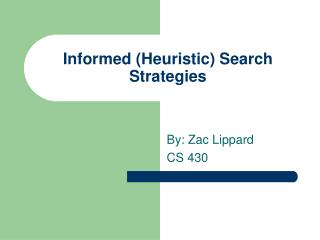DownloadDownload PresentationInformed (Heuristic) Search Strategies

# Informed (Heuristic) Search Strategies

Download Presentation## Informed (Heuristic) Search Strategies

- - - - - - - - - - - - - - - - - - - - - - - - - - - E N D - - - - - - - - - - - - - - - - - - - - - - - - - - -
##### Presentation Transcript

1. Informed (Heuristic) Search Strategies By: Zac Lippard CS 430

2. What is a Heuristic Search? • It is an informed type of strategy • Uses information for problem-solving • Can find solutions faster than uninformed strategies.

3. The 3 Basic Heuristic Search Strategies • Greedy Best-First Search • A* Search • Memory-Bound Heuristic Search

4. Understanding the Search Method • Takes in information in order to find the quickest solution. • Solution should also be efficient. • Not every method will be efficient depending on the algorithms used.

5. Functions Behind the Searches • Evaluation Function • Heuristic Function

6. Evaluation Function • The search algorithm that chooses a node best suited for expansion. • Most functions search for nodes with smallest value.

7. Heuristic Function • The algorithm used to find the cheapest path to the goal node. • Most common form of search algorithm. • Easier to use • More information readily available

8. Greedy Best-First Search • Expands the node that is closest to the goal. • Evaluates nodes by the function:f(n) = h(n) • Example is a straight-line distance heuristic • Uses straight lines with the shortest distance to reach the goal.

9. Problems with this Search • The main problem with this search is even though the algorithm picks shortest distances, it doesn’t necessarily find the shortest distance to the goal.

10. Example of a straight-line distance

11. Greedy Best-First Search Expansion

12. A* Search • Most widely known form of the best-first search • The evaluation function is: f(n) = g(n) + h(n) • g(n) is the cost to reach the node. • The cheapest solution is found by g(n) + h(n)

13. A* Search Cont. • Admissible Heuristic = Optimistic search • It never overestimates; estimates the cost less than actuality. • Contours can be used to find the general range of possible nodes that can be expanded given a certain f-cost.

14. A* Optimality

15. A* Search Example

16. Memory-Bound Heuristic Search • Used to reduce the amount of memory used in the search function. • The IDA*, or iterative-deepening A* algorithm, has a cutoff value of the smallest f-cost from the previous iteration.

17. Recursive best-first search (RBFS) • A better version of IDA*. It regenerates nodes from the same level in order to find the smallest f-cost. • The recursive nature from regenerating the same node over and over is one of the downfalls.

18. Memory-bounded A* (MA*) • This search uses an optimal amount of memory. • A simpler form of MA* is Simplified MA* or SMA*.

19. Example from book • Enhances the RBFS function by removing the highest f-cost node from memory in order to make room for new expanded nodes. • What if there are multiple highest f-cost with the same value? • SMA resolves this by expanding the newest best leaf node and deleting the oldest one.

20. Better Searches • How can we have better searches? • Through meta-level state space. • Meta-level structures allow the computer to capture the internal state of an object-level state (in the books example this would be Romania). • Errors like unnecessary node expansion are interpreted by a meta-level learning algorithm.

21. Conclusion • Types of known search strategies: • Greedy Best-First Search • A* Search • MA* and SMA* • Meta-level Searches • Goal is to always minimize the total cost of the problem.